### Home > PC > Chapter 2 > Lesson 2.3.3 > Problem2-103

2-103.
1. Given the graph of f(x), sketch the following functions. Homework Help ✎

1. f(x)

2. f(x + 2) − 1

3.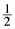f(x)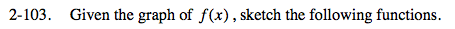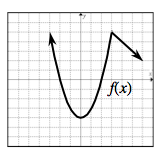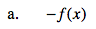The negative gives all of the f(x)-values the opposite sign.
What does this do to the graph?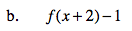The 2 and –1 shift the graph. Which directions?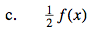All of the original function values are halved.